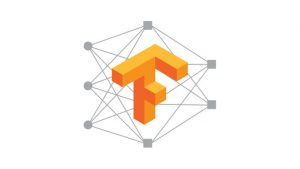Want to share your content on R-bloggers? click here if you have a blog, or here if you don't.Deep learning is under active development. Papers with new approaches are being published every day. In this set of exercises we will go through some of the newer methods that boost the neural network’s performance. By the end of this post, you will be able to train neural networks with adaptive learning rates and apply methods to avoid (overfitting)[https://en.wikipedia.org/wiki/Overfitting], it is recommended to check out the following tutorials before start solving the exercises. : basics part 1, basics part 2,
regression analysis,
classification analysis.
Moreover, a great overview of the algorithms that we will go through at this tutorial can be found here, it is highly recommended to go through this post. It is very likely to be one of the best guides of adaptive learning methods out there.

We will use the ‘mtcars’ built-in dataset for this post. The data set is easy to be trained so we will not use a formal evaluation metric(accuracy) but we will plot the logistic regression so that you can really see the impact of each individual optimization technique.

Before proceeding, it might be helpful to look over the help pages for the `tf\$placeholder`, `tf\$zeros`,`tf\$multiply`, `tf\$add`, `tf\$global_variables_initializer`, `tf\$Variable`, `tf\$sigmoid_cross_entropy_with_logits`, `tf\$reduce_mean, tf\$train\$GradientDescentOptimizer`,
`tf\$train\$MomentumOptimizer`, `tf\$train\$AdamOptimizer`, `tf\$train\$AdadeltaOptimizer`, `tf\$train\$AdadeltaOptimizer`, `tf\$train\$RMSPropOptimizer`, `tf\$train\$AdamOptimizer`.

Answers to the exercises are available here. If you obtained a different (correct) answer than those listed on the solutions page, please feel free to post your answer as a comment on that page.

Exercise 1

Split the data set into training and testing set. The 80% should be the training data and the rest 20% should be the test data.
Create the placeholders, the parameters (initialize them at 0), the initialization operation, the logit, and evaluate the model using mean cross entropy.

Exercise 2

Train the model using gradient descent algorithm with learning rate 0.01. Plot the results and see how it performs.

Exercise 3

Train the model using momentum update algorithm with learning rate 0.01 and momentum of 0.1. Plot the results and see how it performs.

Exercise 4

Train the model using momentum update algorithm with learning rate 0.01 and momentum of 0.9. Plot the results and see how it performs.

Exercise 5

Train the network using the nesterov momentum update. Does it perform better?

Exercise 6

Use the Adagrad algorithm with learnign rate 0.01, beta1 term 0.9, beta2 term 0.999 and epsilon 1e-08 (recommended hyperparameters). Bear in mind that Adagrad is considered to be a very aggressive algorithm.

Exercise 7

Use the Adadelta algorithm with learnign rate 0.01, decay term 0.1 and epsilon 1e-08.

Exercise 8

Use the Adadelta algorithm with learnign rate 0.01, decay term 0.9 and epsilon 1e-08.

Exercise 9

Use the RMSprop algorithm with learnign rate 0.01, decay 0.9, momentum term 0.1 and epsilon 1e-10.

Exercise 10

Use the Adam algorithm with learning rate 0.01, decay 0.9, momentum term 0.1 and epsilon 1e-10.

Disclaimer: Plotting is not a best practice to test the goodness of fitness, but we do it because it is a very simple way to see the difference and helps you understand the difference betweeen the models.